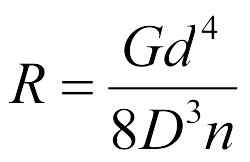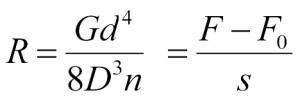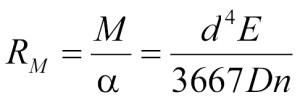The spring rate, also called spring constant or spring hardness, depends on the spring material and the shape of the compression spring, extension spring or torsion spring. The formula for calculating the spring rate for cylindrical springs made of round wire with a linear spring characteristic is as follows:

Compression spring Calculation of spring rate or spring constant (N/mm) Compression spring formularyTension spring Calculation of spring rate, or spring constant (N/mm) Collection of formulas for tension springsTorsion Spring Calculation Spring Moment Rate (Nmm)(Formulary Torsion Springs)Overview of the spring materials

∝ = angle of rotation (°)
d = wire diameter (mm)
D = mean coil diameter (mm)
E = modulus of elasticity (N / mm²)
F = spring force (N)
M = torque (Nmm)
s = spring deflection (mm)
n = resilient coils (n)
G = sliding or shear modulus (N / mm²)